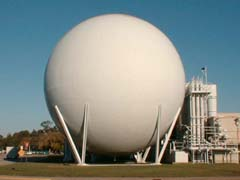Ch 7. Stress Analysis Multimedia Engineering Mechanics PlaneStress PrincipalStresses Mohr's Circlefor Stress Failure PressureVessels
 Chapter 1. Stress/Strain 2. Torsion 3. Beam Shr/Moment 4. Beam Stresses 5. Beam Deflections 6. Beam-Advanced 7. Stress Analysis 8. Strain Analysis 9. Columns Appendix Basic Math Units Basic Equations Sections Material Properties Structural Shapes Beam Equations eBooks Dynamics Fluids Math Mechanics Statics Thermodynamics Author(s): Kurt Gramoll ©Kurt GramollChapter 7 Stress AnalysisTopics Covered Plane Stress Principal Stresses and Maximum Shear Stresses Mohr's Circle for Stress Failure 3D Stress State (under development) Pressure Vessels Stress Concentrations (under development) The topic menu above allows you to move directly to any of the four sections for each topic. The sections are: Case Intro: To help introduce and understand the basic principles, a case study is presented. Theory: This section will review the basic principles and equations that you should know to answer the exam questions. It does not give detailed derivations of the theory. Case Solution: The case study is solved in detail in this section. Graphics, narrations, animations, and equations are used to help you understand how the problem was solved. Example: Addition example problem(s) for some sections.